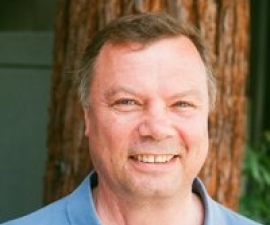### Research Expertise and Interest

mathematics, representation theory, mathematical physics, low-dimensional topology

### Research Description

Nicolai Reshetikhin's research interests lie at the interface of mathematical physics, geometry and representation theory, more specifically in quantum field theory, statistical mechanics, geometry and low-dimensional topology, and representation theory of quantum groups. Representation theory of quantum groups and of quantized universal enveloping algebras is the main algebraic structure behind integrability of most known "non-Gaussian" integrable models in classical and quantum mechanics, in field theory, and in statistical mechanics. This direction has many fascinating problems ranging from answering deep questions in statistical mechanics (in solvable examples, where more tools are available) to deep structural results in representation theory which are motivated by physical applications. Representation theory of quantum groups is also a powerful tool behind constructions of invariants of knots and 3-dimensional manifolds. Invariants of knots and 3-manifolds can also be obtained by quantizing classical topological field theories. Such theories are, as a rule, are gauge invariant (examples are Chern-Simons theory, BF theory, Poisson sigma model and others). Quantization of such theories involve a lot of modern geometry. One of the challenges in this direction is to develop semiclassical quantization of such theories for space time manifolds with boundary.